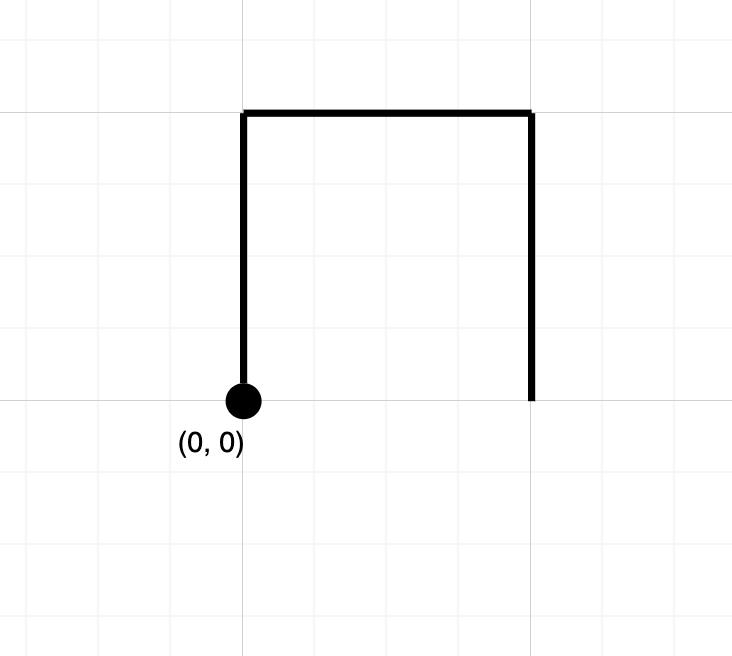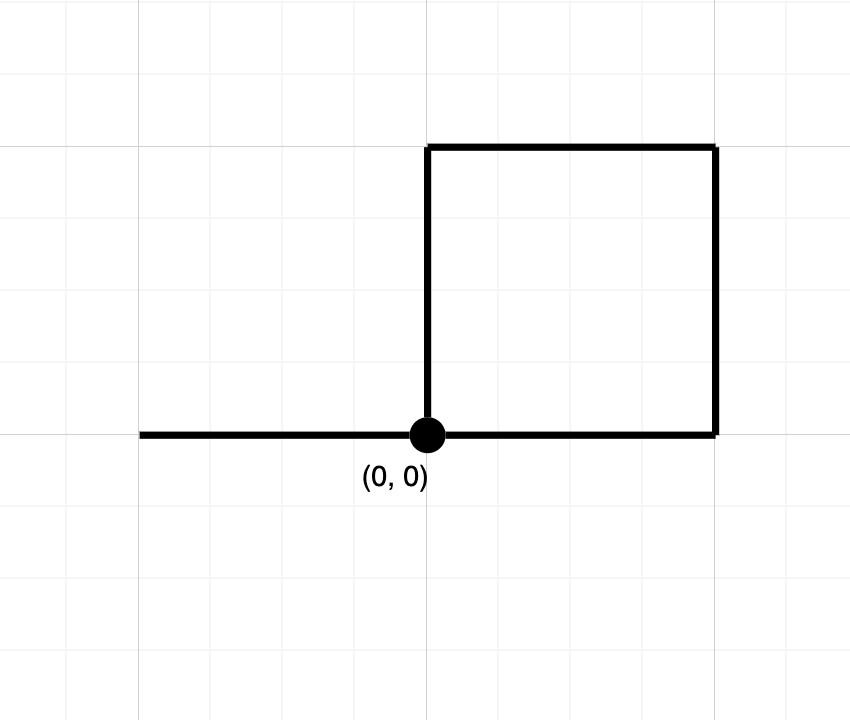1496. Path Crossing

#### QUESTION:

Given a string path, where path[i] = ‘N’, ‘S’, ‘E’ or ‘W’, each representing moving one unit north, south, east, or west, respectively. You start at the origin (0, 0) on a 2D plane and walk on the path specified by path.

Return true if the path crosses itself at any point, that is, if at any time you are on a location you have previously visited. Return false otherwise.

Example 1:``````Input: path = "NES"
Output: false
Explanation: Notice that the path doesn't cross any point more than once.
``````

Example 2:``````Input: path = "NESWW"
Output: true
Explanation: Notice that the path visits the origin twice.
``````

Constraints:

``````1 <= path.length <= 104
path[i] is either 'N', 'S', 'E', or 'W'.
``````

#### SOLUTION:

``````class Solution {
fun isPathCrossing(path: String): Boolean {
var visited = ArrayList<Pair<Int,Int>>()
var start:Pair<Int,Int> = Pair(0,0)
for (p in path) {
when (p) {
'N' -> {
start = start.copy(second = start.second + 1)
}
'S' -> {
start = start.copy(second = start.second - 1)
}
'E' -> {
start = start.copy(first = start.first + 1)
}
'W' -> {
start = start.copy(first = start.first - 1)
}
}
var tmp = Pair(start.first, start.second)
if (visited.contains(tmp)) return true